# Binomial Theorem - Exercise 2.3 : Class 12 Math

Binomial Theorem - Exercise 2.2 : Class 12 Mathematics. Complete Binomial Theorem Exponential and Logarithmic Series Exercise 2.2 of Class 12 Math NEB

## Chapter 2: Binomial Theorem.

### Exercise: 2.3

Complete Exercise of Binomial Theorem - Exercise 2.3 : Class 12 Mathematics 2080 NEB.

Exercise 2.3, Is about Exponential and Logarithmic Series.

Read: NEB Class 12 Mathematics All Chapter Exercise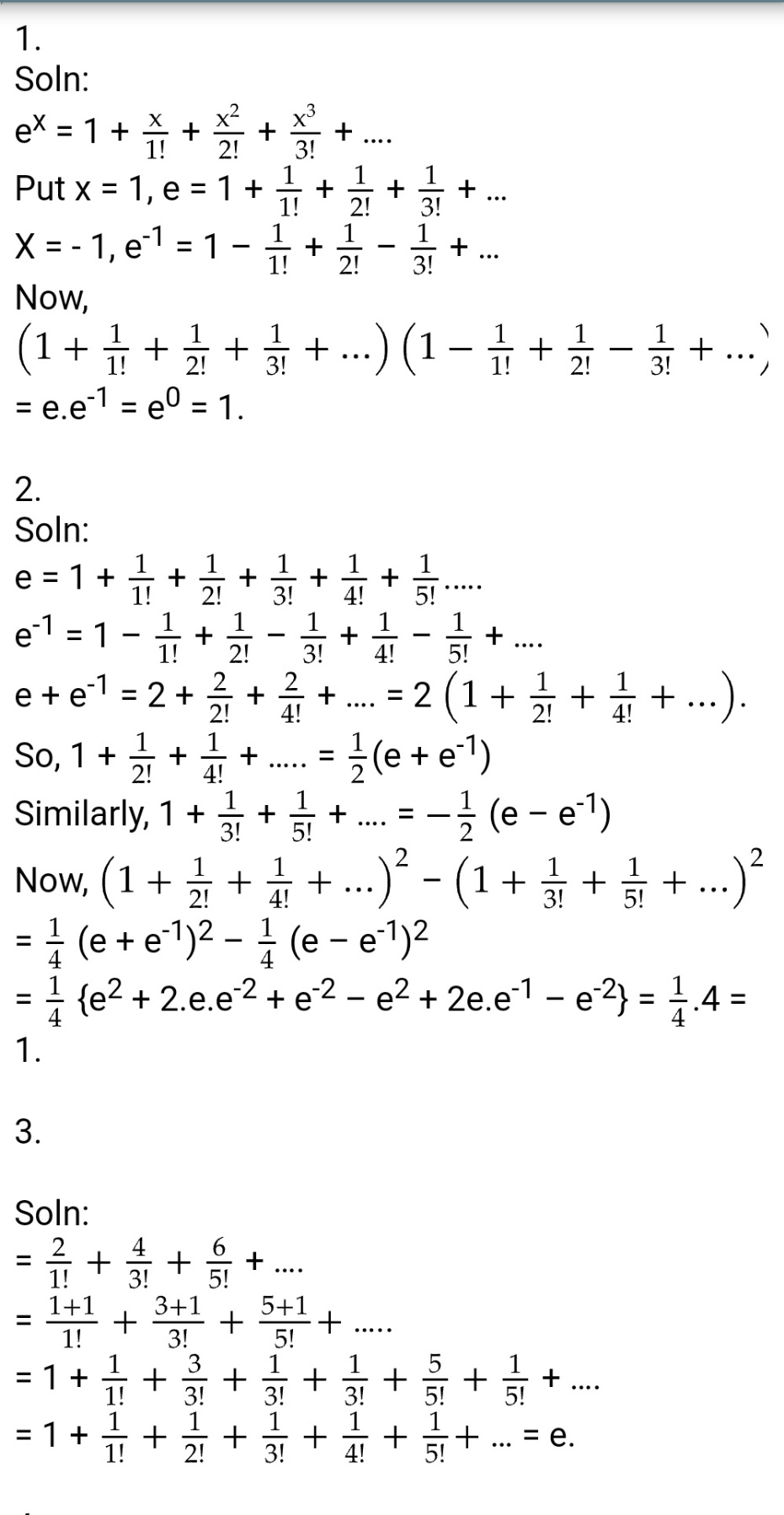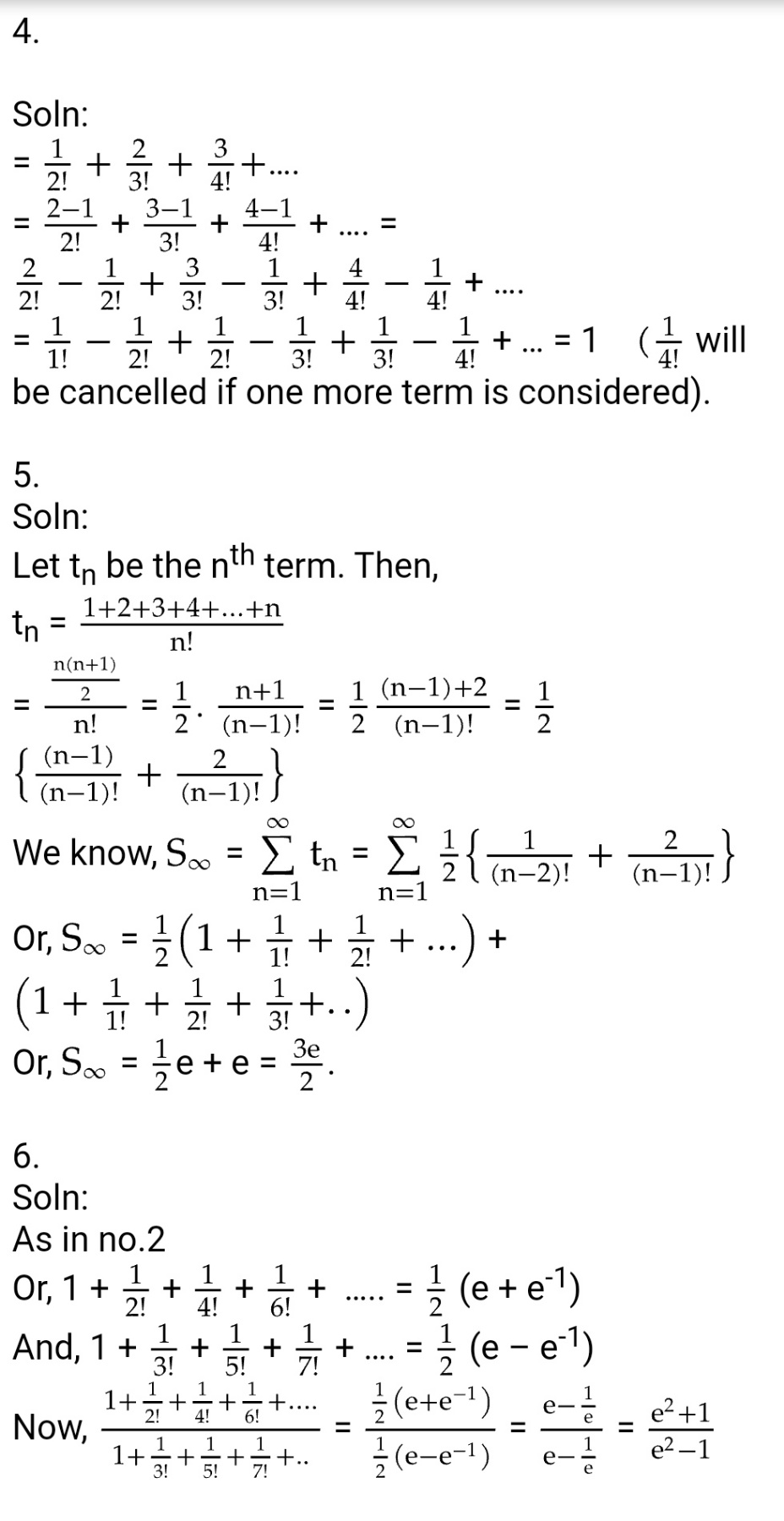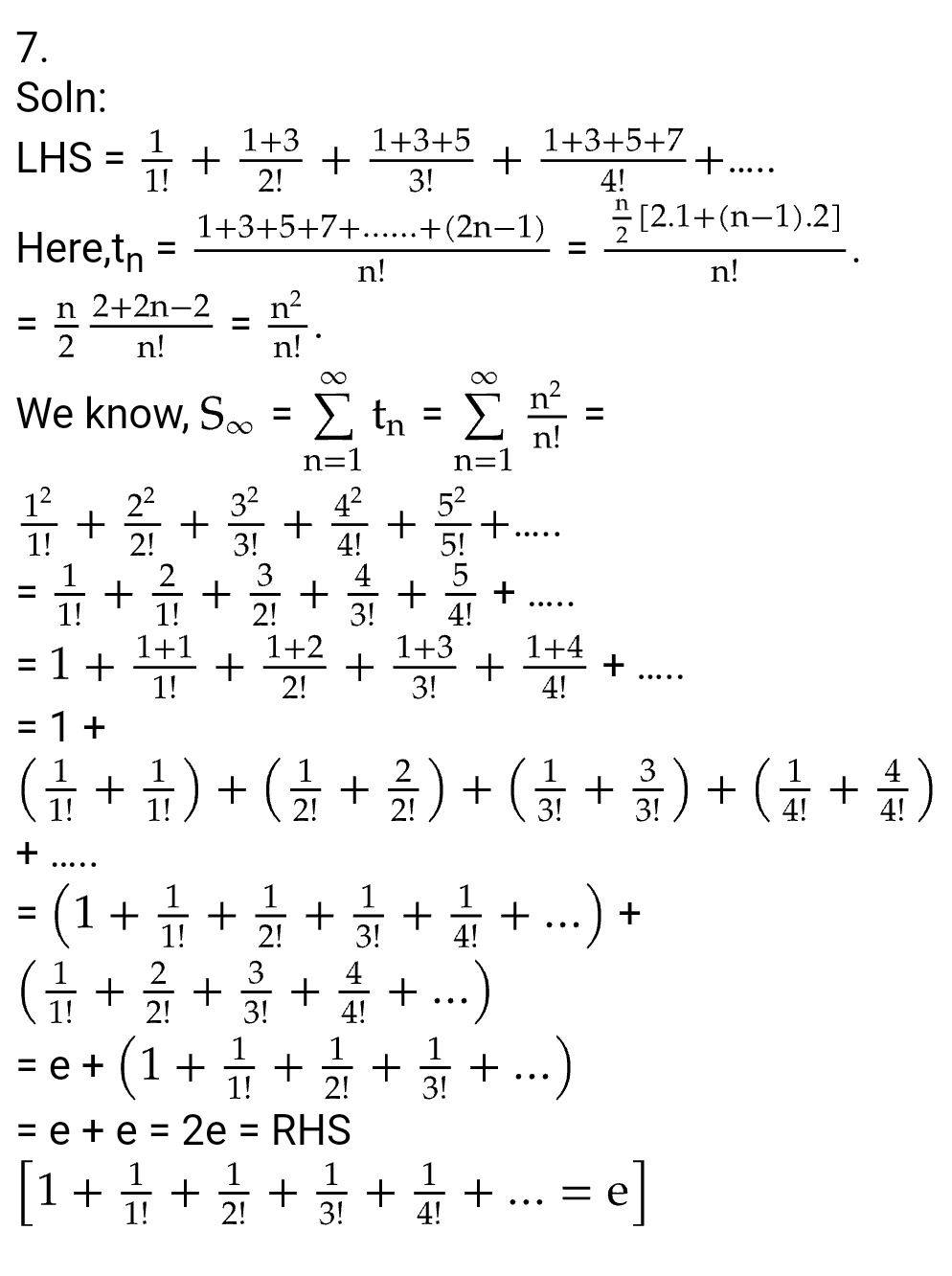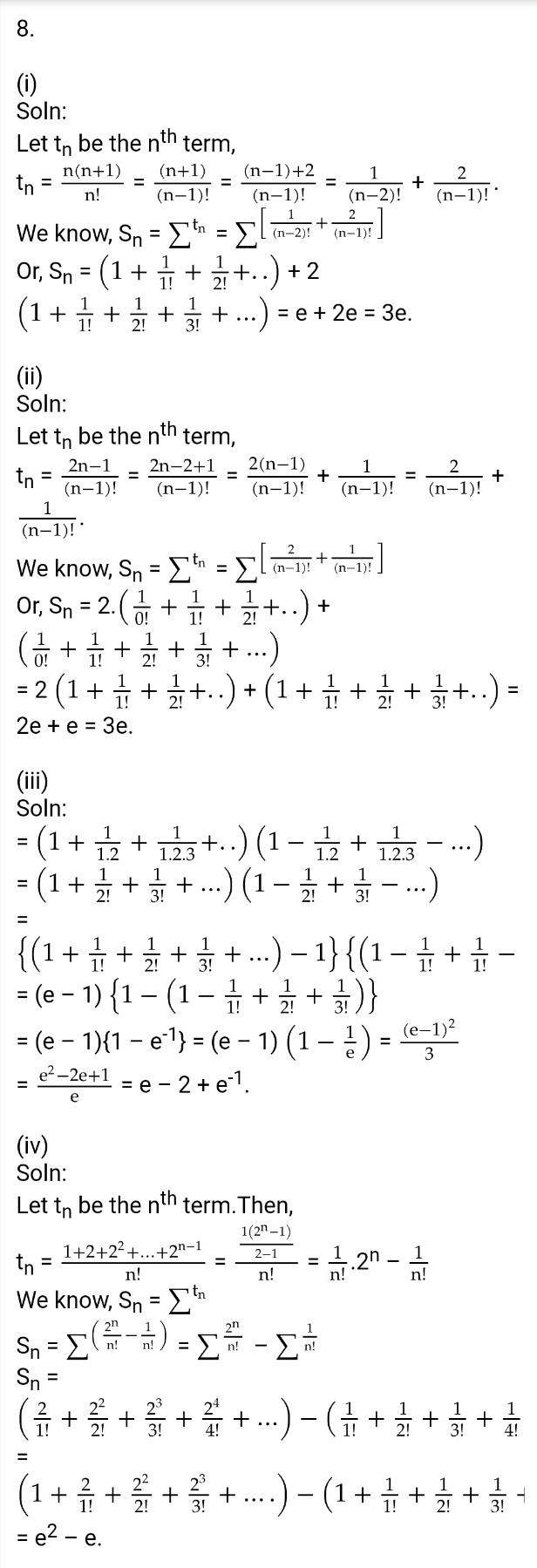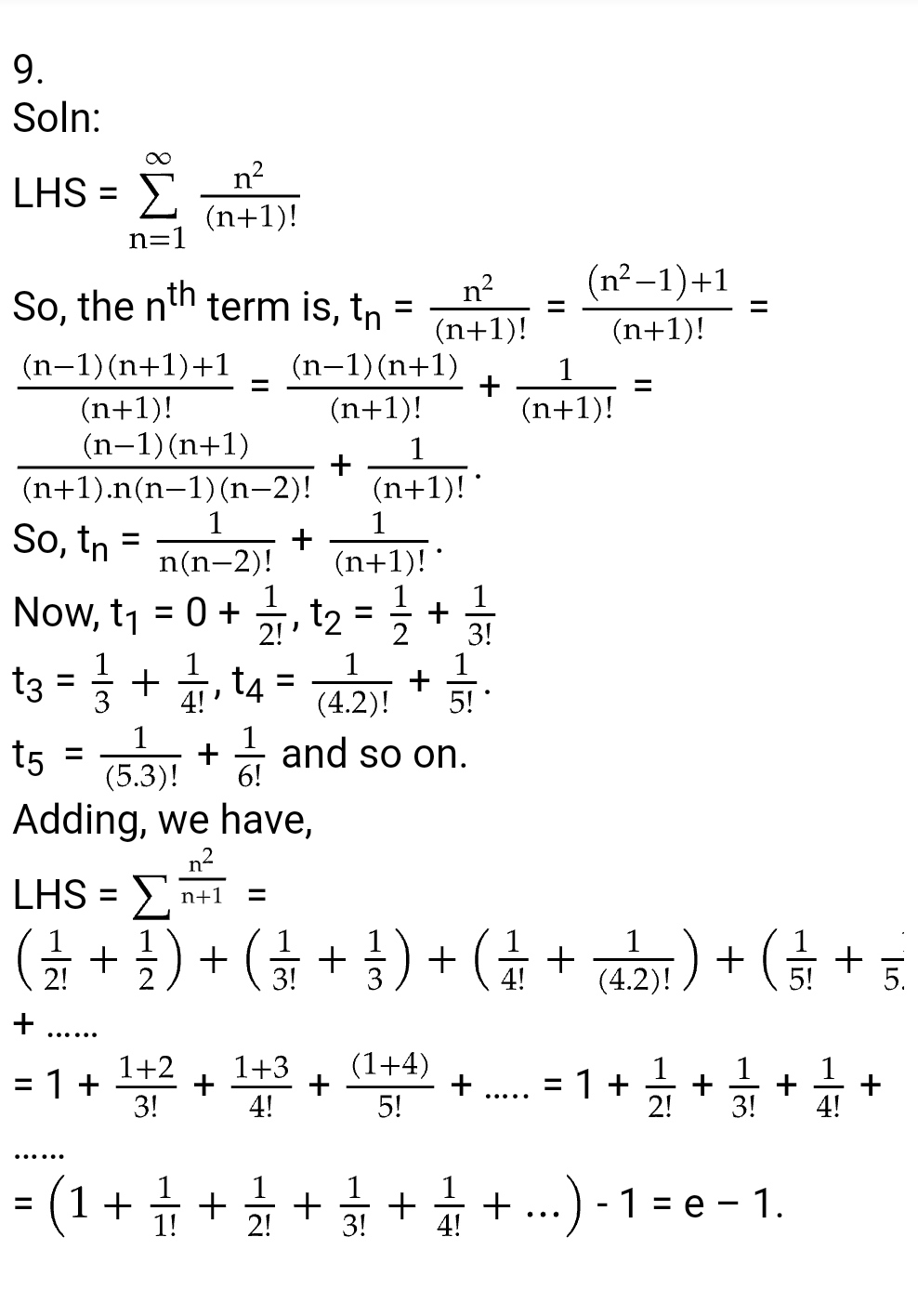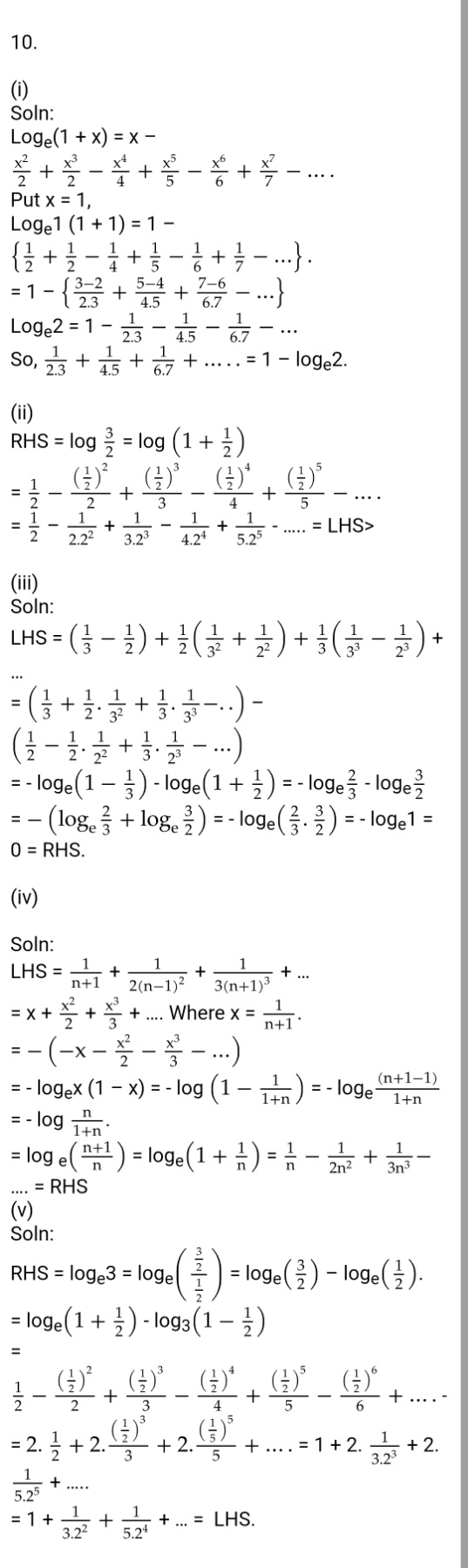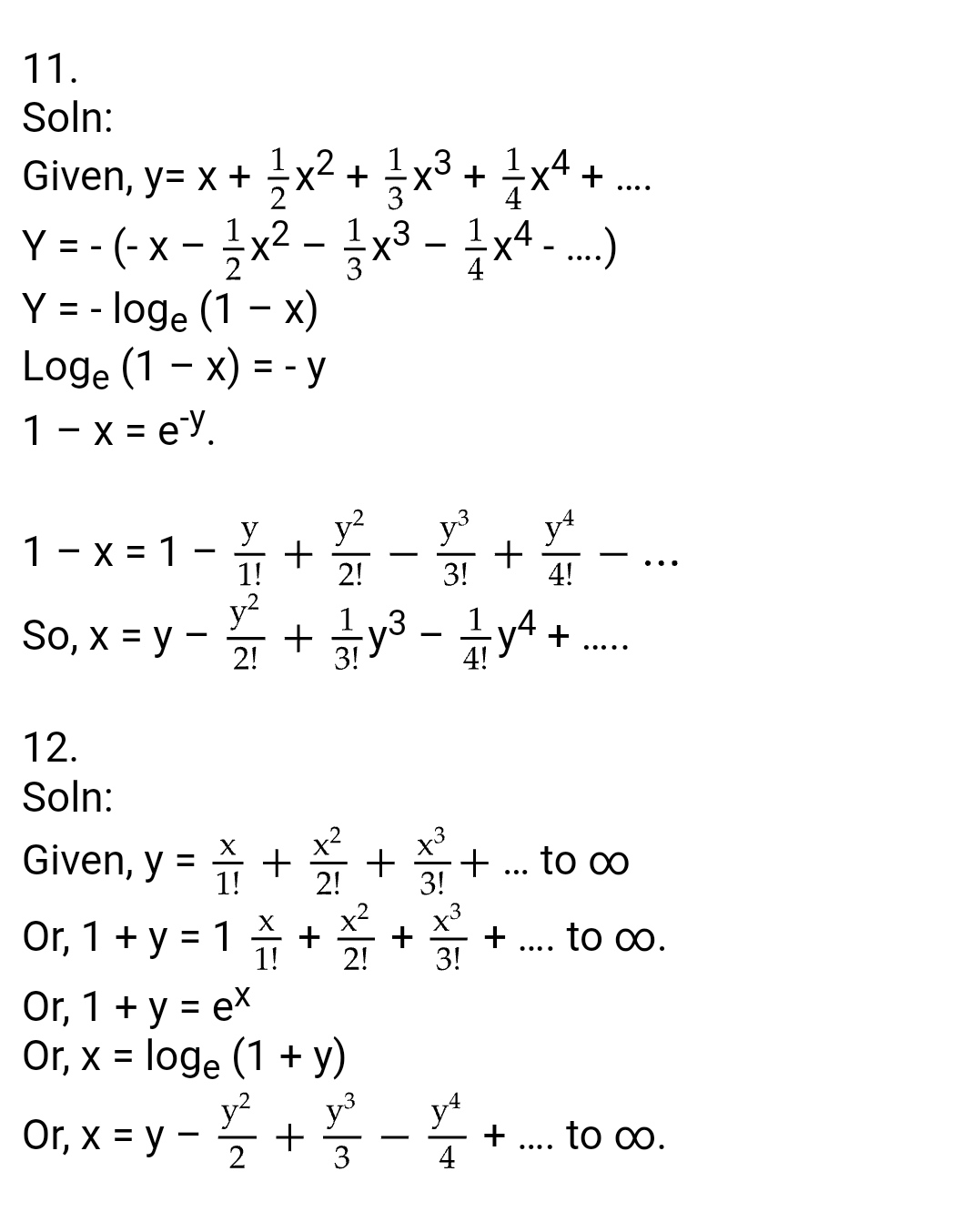Binomial Theorem - Exercise 2.1 : Class 12 Math

Binomial Theorem - Exercise 2.2 : Class 12 Math

Getting Info...